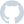# W

Ward279 stars, 18 forks

# Using with Hypothesis

You can use Hypothesis with Ward out of the box.

Hypothesis must be installed separately:

\$
pip install hypothesis

When using Hypothesis with Ward, you must use the `@using` decorator in order to inject fixtures into tests. Hypothesis does not support tests with default arguments.

Here's an example of a FAILing test that uses Hypothesis. It makes the rather strange assertion that every list of integers contains the integer `1`, which obviously isn't true, but provides us an example of how Hypothesis works.

`from hypothesis import givenfrom hypothesis.strategies import lists, integersfrom ward import test@test("every list of integers contains the number 1")@given(l=lists(elements=integers()))def _(l):    print(l)  # Let's print out the list to see what's happening!    assert 1 in l`

Hypothesis calls the test above multiple times. Each time it calls it, it passes a different list of integers into the test. Each of these calls are known as "examples". We don't know in advance how many examples Hypothesis will run, because it generates them at runtime, and the generated examples are dependent on each other.

Let's run this test:

\$
ward

Here's the output produced when the test runs:

As you can see, the test was called by Hypothesis multiple times, passing in a different list of integers each time. The final line of captured output was written by Hypothesis itself, and it tells us the first falsifying example Hypothesis found. That is, the first example it generated which resulted in the FAIL.

Deeper Hypothesis integration is planned for future versions of Ward, including the ability for fixtures to be regenerated for each Hypothesis example.